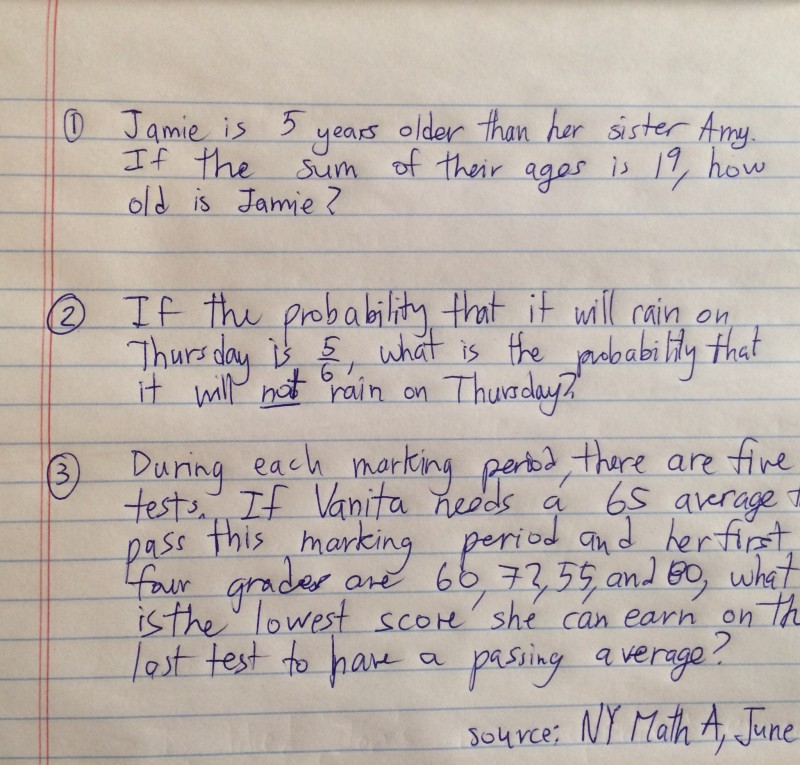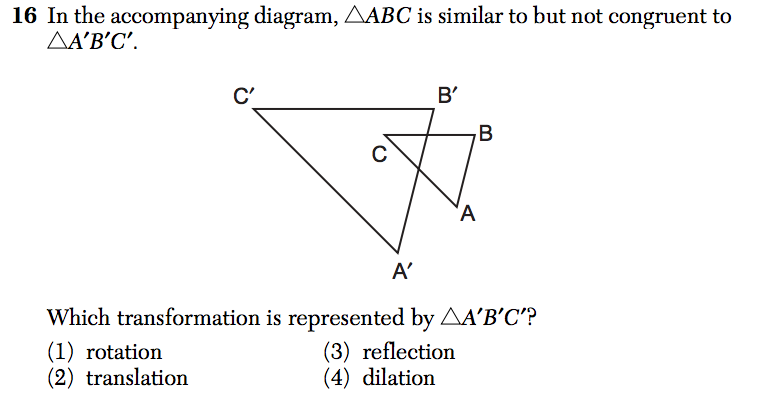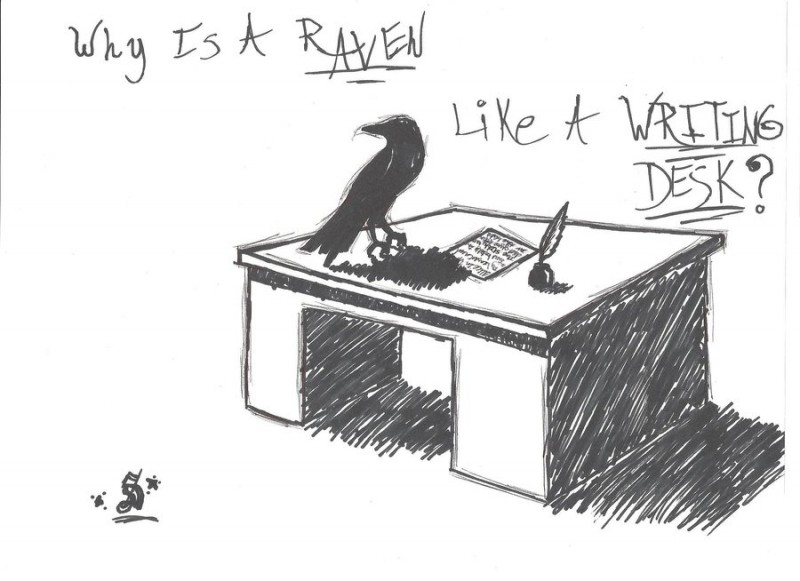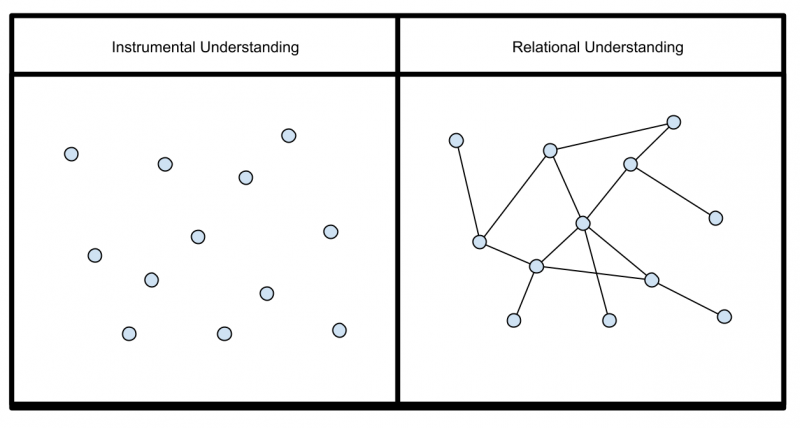## The Reflective Educator

### Education ∪ Math ∪ Technology

CloseIs this curriculum?

On the day before I first started teaching, the district coordinator came to me and handed me a piece of paper with twenty questions on it. “Here’s what you have to teach, David. If your students can answer all twenty of these questions by the end of the year, you will be fine.

Needless to say that this was insufficient curriculum. But what kind of curriculum would have been useful for me at that stage in my career?

A place many math educators, especially in our accountability-driven system, seem to start when teaching mathematics is teaching children how to solve questions. “Here’s how you solve this kind of question.” The connections between different problems are left for students to discover on their own.Strategy: If the triangles are a different size, the transformation is a dilation.

The advantage to this system is that you can look at the state assessment and check off of all of the question types and feel like you have done your job. For the old NY state exam, this approach works in the sense that students were able to sit down at the exam and feel like they had been prepared for every question since the assessment was so predictable, even if they didn’t always know how to solve the problems .

The problem with this approach is that students now have to remember each question archetype and each solution to each type of problem separately, leading to a relatively unorganized and overwhelming set of problem-solving schema for students. This leads to students forgetting how to solve individual problems, forgetting which solution strategy they should use when, or misapplying strategies to solve problems for which the strategy is not appropriate. Even when students do master all of the problem types, knowing how to solve problem x, y, or z doesn’t help students make connections when they start studying further areas of mathematics.

To their credit, every textbook author I’ve ever read takes a different approach (to some degree). Textbooks start by dividing the year’s worth of mathematics to be learned into units of study and apportioning mathematical principles into those units. Within each unit of study, specific problems are used to illuminate mathematical ideas and ideally students at the end of a unit can articulate the mathematical ideas they have learned, rather than just the problems to which they apply. Where textbooks often fall down is in making the connections between units and ideas explicit.

When well done, problems become vehicles for teaching mathematical principles. Mathematical representations (like graphs, tables, etc…) are embedded across the units so that students get multiple exposures to these critical representations and can use them to make sense of similarities and differences between different mathematical ideas. Ideally students explicitly learn connections between different mathematical ideas so that they see, for example, how solving a linear equation is related to solving a quadratic equation and how the graph of an absolute function is related to the graph of a linear function.One way to support students in making these connections is to ask them to answer reflection questions like: How is what we learned today related to what we learned yesterday? How is this problem we solved today like the problems we solved last week? What did we learn today that we can probably generalize and use to solve other problems? At the very least while planning, make sure you can answer these questions yourself.

A major disadvantage of the second approach to teaching mathematics is that it takes much more work to organize curricular resources into the general themes and to make the connections between ideas explicit. Rare is the classroom teacher who has time to do this all herself. This is one of the reasons why I think well-organized curriculum of some kind is always going to be a helpful resource.There is a gradient between the two approaches to teaching mathematics.

For further reading on a related topic, check out this post on instrumental versus relational knowledge.